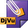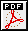# Dimitry Leites - Publications

[Books and chapters in books] [Books edited] [Books translated] [Papers] [Encyclopedia entries] [Workouts of SoS]
* if with co-authors and co-editors

[E1]  F. Berezin, Introduction to superanalysis. Edited and with a foreword by A.A. Kirillov. With an appendix by V.I. Ogievetsky. Translated from the Russian by J. Niederle and R. Kotecký. Translation edited by D. Leites. Mathematical Physics and Applied Mathematics, 9. D. Reidel Publishing Co., 1987, xii+424 pp. MR 89b:58006Russian original: F. Berezin, Introduction to algebra and analysis with anti-commuting variables. Moscow State University, 1983. MR 86a:58006[E2]  F. Berezin, Lectures on statistical physics. 2nd edition, revised and edited by D. Leites, with editor's preface. MCCME, Moscow, 2008, 196 pp. (in Russian).

[E3*] Recollections on Felix Alexandrovich Berezin - the founder of supermathematics. Compiled by E.G. Karpel and R.A. Minlos, edited by D. Leites and I. Tyutin. MCCME, Moscow, 2009, 382 pp. (in Russian).[E4]  F. Berezin, Lectures on statistical physics. Revised and edited by D. Leites. Translated into English and edited by D. Leites. A. Salam School of Mathematical Sciences, Lahore, 2009, 183 pp.[Book cover]

[E5]  A. Kirillov, J. Bernstein, V. Arnold, Representation theory. Vol. 1. Representations of finite and compact groups. Representations of simple Lie algebras. Edited by D. Leites. A. Salam School of Mathematical Sciences, Lahore, 2009, 122 pp.[Book cover]

[E6*] Yu. Manin, Introduction to the theory of schemes and quantum groups. Revised and edited by D. Leites and S. Lvovsky. MCCME, Moscow, 2012, 256 pp. (revised version of earlier editions of several books; in Russian).

[E7]  F. Berezin, Introduction to superanalysis. Revised and edited by D. Leites and with appendix "Seminar on Supersymmetry. Vol. 1½". MCCME, Moscow, 2013, 432 pp. (in Russian).[E8*] J. Bernstein, S. Bouarroudj, B. Clarke, P. Grozman, A. Lebedev, D. Leites, I. Shchepochkina, Representation theory. Vol. 2. Nonholonomic distributions in representation theory. Quest for simple modular Lie algebras and Lie superalgebras. Edited by D. Leites. A. Salam School of Mathematical Sciences, Lahore, 2009, 536 pp.   Draft:[Book cover]

### In preparation, available in a preliminary form

[E9*] The 60-odd years of the Moscow Mathematical Olympiads. Compiled with A. Shapovalov and P. Grozman. Edited by D. Leites, ca. 800 pp.

[E10] Yu. Manin, Introduction to the theory of schemes. Revised and edited by D. Leites, 182 pp. (revised version of earlier editions of several books).[E11] V. Sergeev, The thermodynamic approach to markets. With appendix by A. Vershik, ca. 200 pp. MPIMiS preprint 76/2006; arXiv:0803.3432.

[E12] V. Prasolov, Encyclopedia of problems in plane and solid geometry, ca 900 pp.

[E13] V. Prasolov, Geometric problems of Ancient World, ca 120 pp.